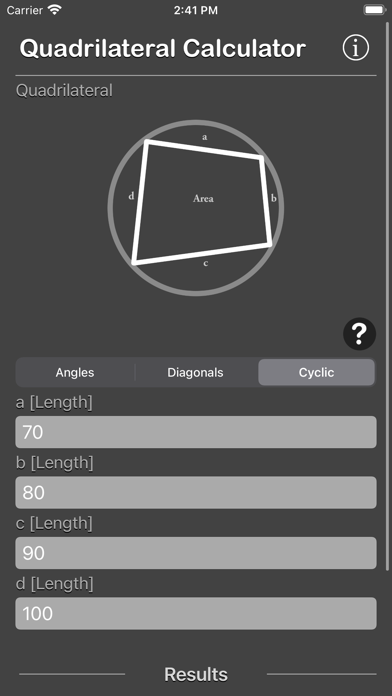## Quadrilateral Calculator - Lumos Educational App StoreNA1
Price -\$2.99
\$2.99

#### DESCRIPTION:

Quadrilateral Calculator calculate Area and Perimeter from difference type of Quadrilateral. This is a efficient and accurate tool for calculating land area or measurement of land. Or to determine rough square footage of various irregularly shaped storage closets. Features: - Calculates the area and perimeter of a quadrilateral given four sides and two opposite angles. - Calculates the area and perimeter of a quadrilateral given four sides and two diagonals. - Calculates the area and perimeter of a quadrilateral inscribed in a circle given four sides. - Result are calculate on the fly - Incl

#### OVERVIEW:

Quadrilateral Calculator is a free educational mobile app By Nitrio.It helps students practice the following standards .

Developer: Nitrio

Developer URL: http://www.nitrio.com

Software Version: 1.1

Category: Utilities

Release Date: 2018-05-19T14:29:26Z#### Are you the Developer?EdSearch WebSearch